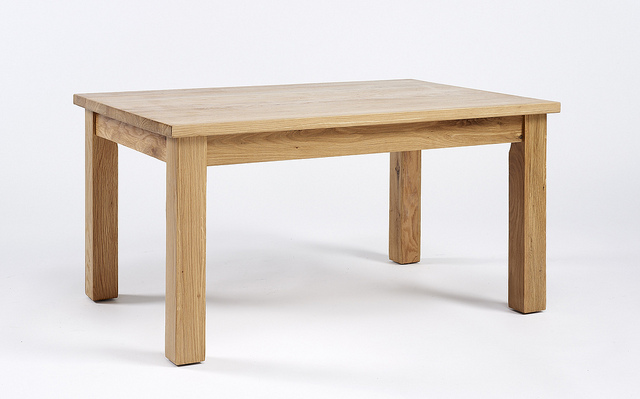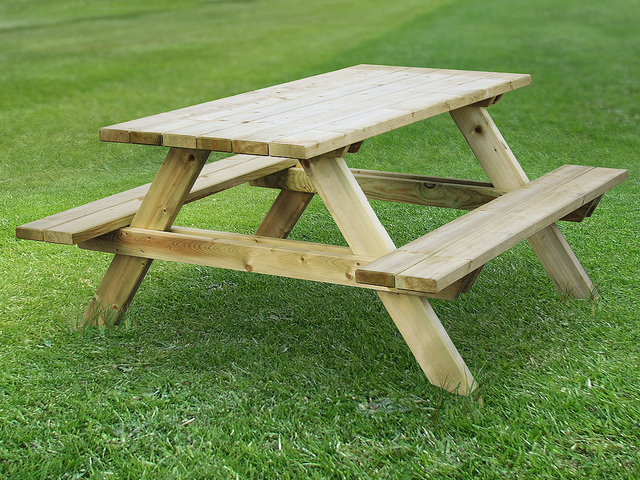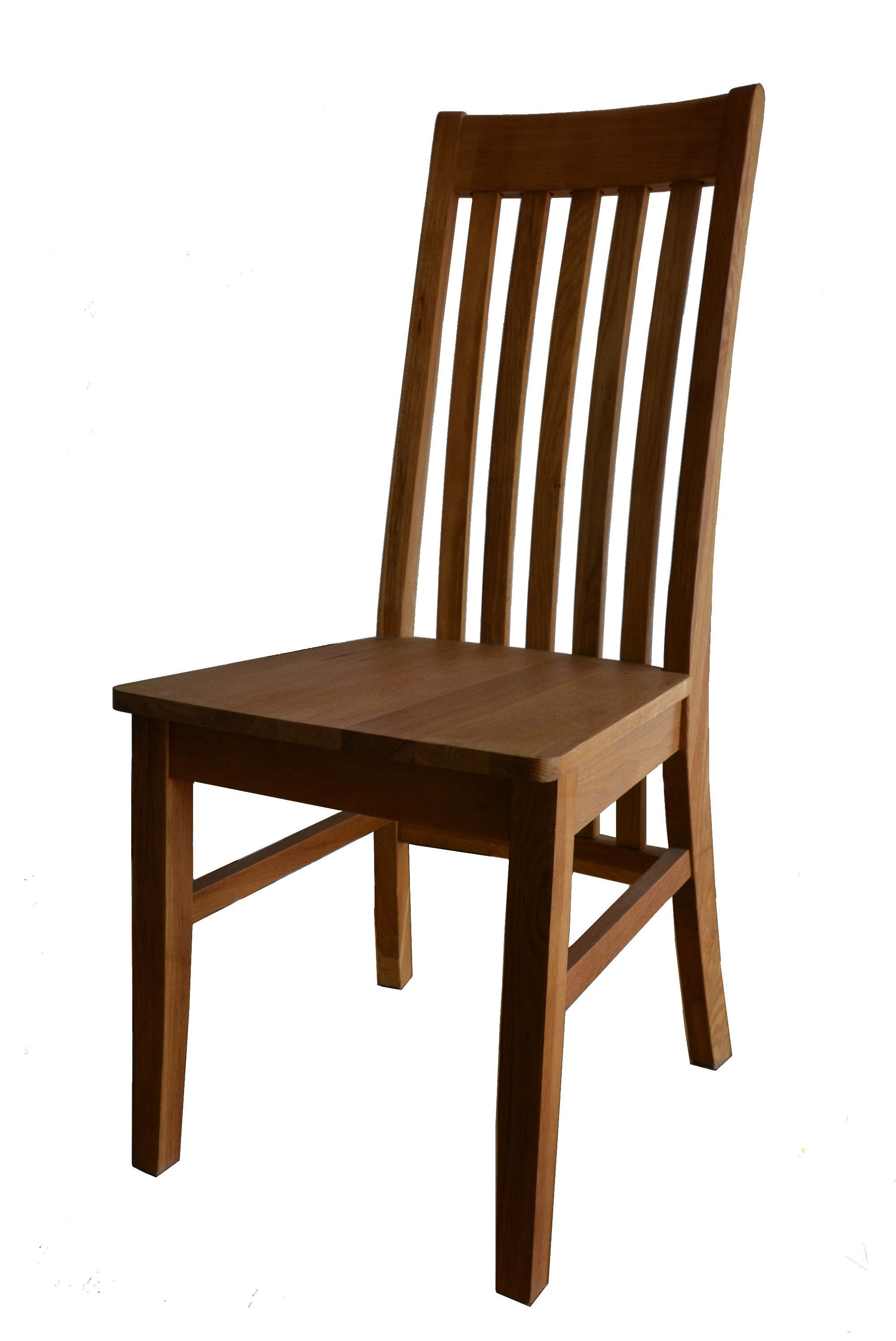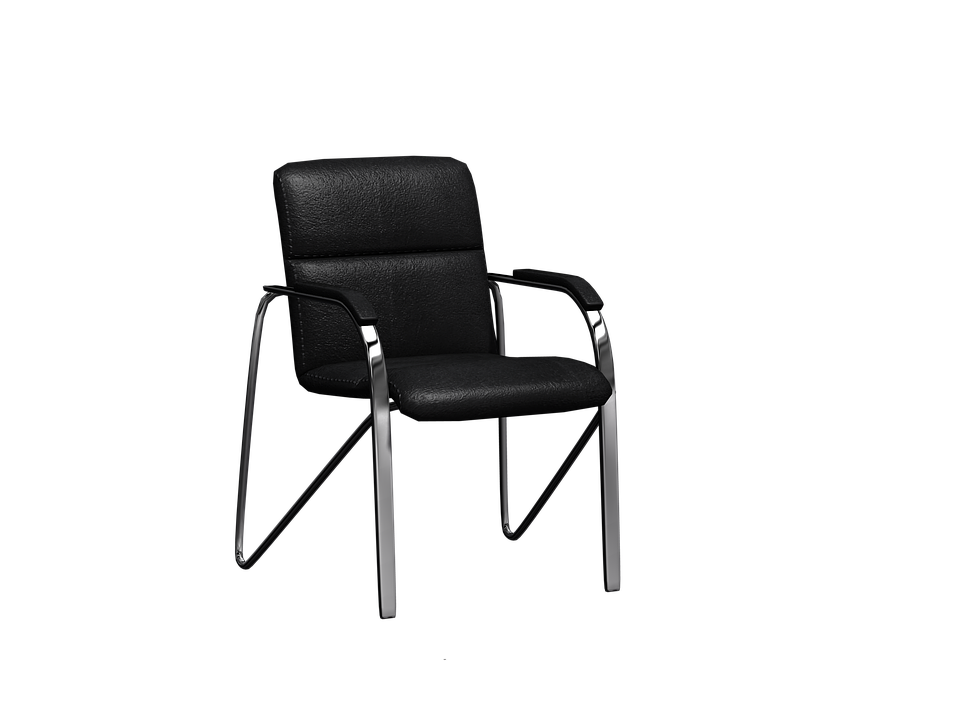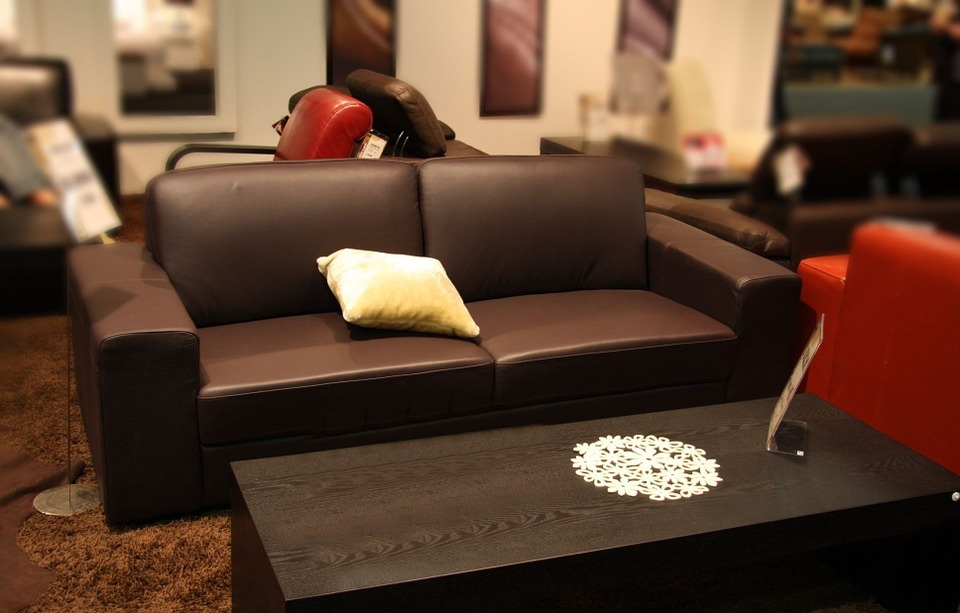# Domain Specific Languages in Clojure

## Lingua Franca

/ˌlɪŋɡwə ˈfraŋkə/
noun

A language that is adopted as a common language between speakers whose native languages are different.
“a problem well put is half solved.” John Dewey

## What Is a DSL Good For?

• We use DSL's to state the problem we are trying to solve explicitly & correctly.
“When concepts are integrated into a wider one, the new concept includes all the characteristics of its constituent units; but their distinguishing characteristics are regarded as omitted measurements, and one of their common characteristics determines the distinguishing characteristic of the new concept: the one representing their ‘Conceptual Common Denominator’ with the existents from which they are being differentiated.” Ayn Rand (ItOE)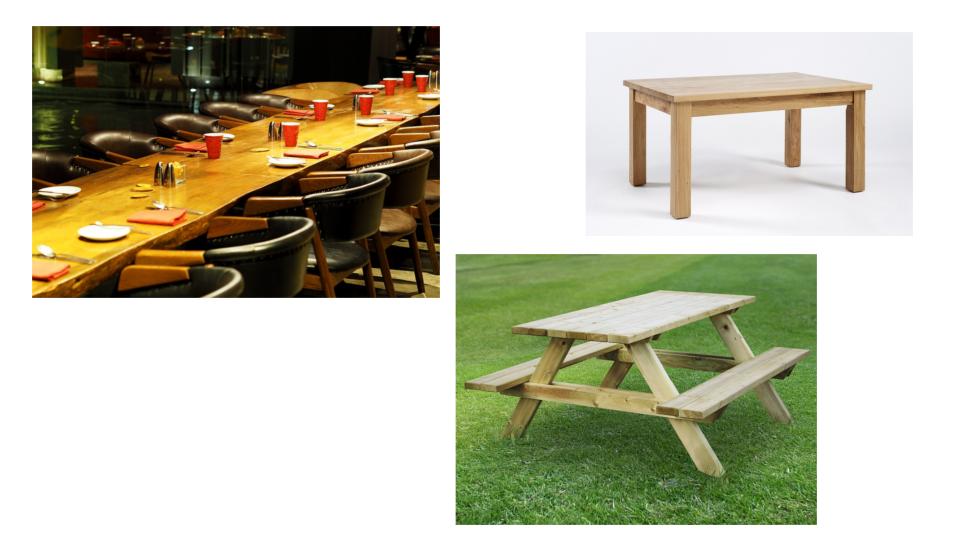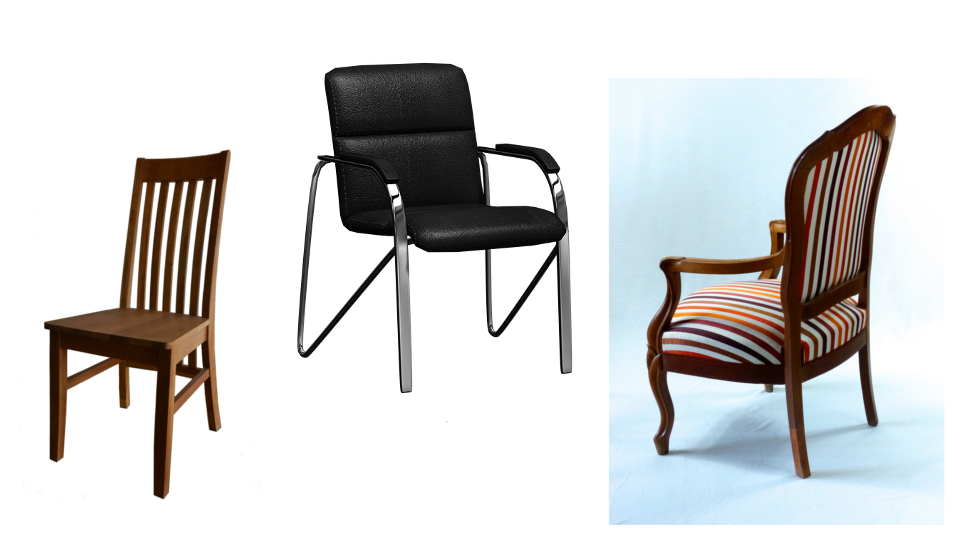## What Is a DSL Good For?

• We use DSL's to state the problem we are trying to solve explicitly & correctly.
• We use DSL's to hide implementation details.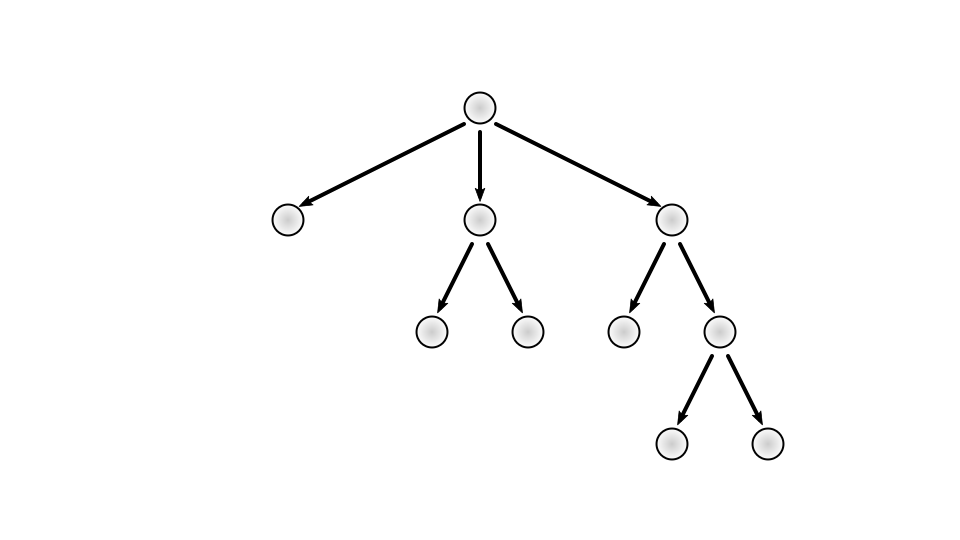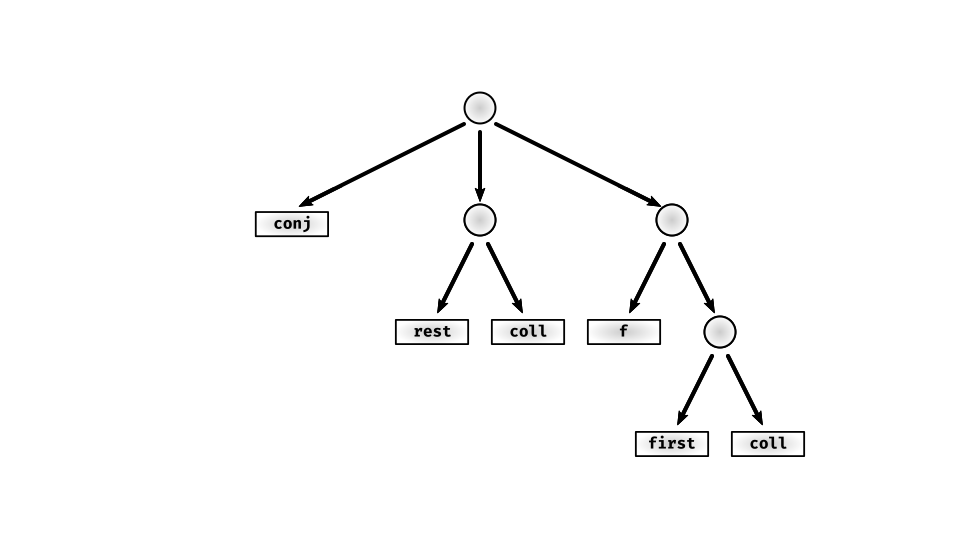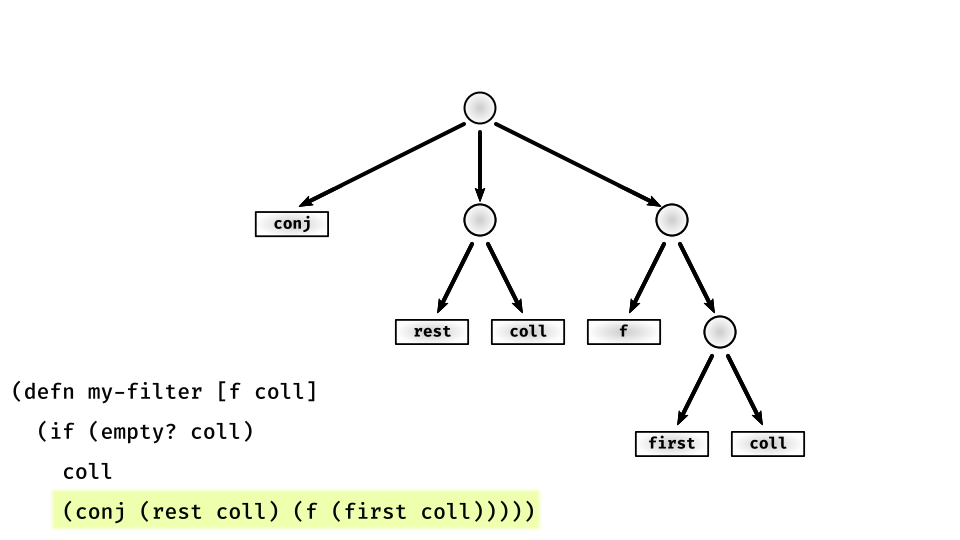## Independent vs Embedded

• An embedded DSL runs within the host environment. As a result host language is fully (or mostly) available.
• An independent DSL runs above the host environment. Therefore host language is not directly available. All interaction must be performed through declared interfaces. This opacity also means the host environment can be replaced without any changes to the independent DSL.

#### Independent DSL Example: Instaparse

``````
PROGRAM = (WHITESPACE | COMMENT | APPLICATION)*
APPLICATION = "(" (WHITESPACE | ATOM | LIST | APPLICATION)* ")"
<ATOM> = SYMBOL
(* <ATOM> = BOOLEAN | NUMBER | STRING | SYMBOL *)
(* BOOLEAN = "True" | "False" *)
COMMENT = #";;\p{Blank}*" #"[^\s]?[^\n]*" "\n"
LIST = "[" (WHITESPACE | ATOM | LIST | APPLICATION)* "]"
(* NUMBER = #"-?\d+(\.\d+)?" *)
(* STRING = (<"\""> #"[^\"]* <"\"">) | (<"'"> #"[^']* <"'">) *)
SYMBOL = #"\w[\w\d-]*"
WHITESPACE = #"\s+"
``````

## Shape Language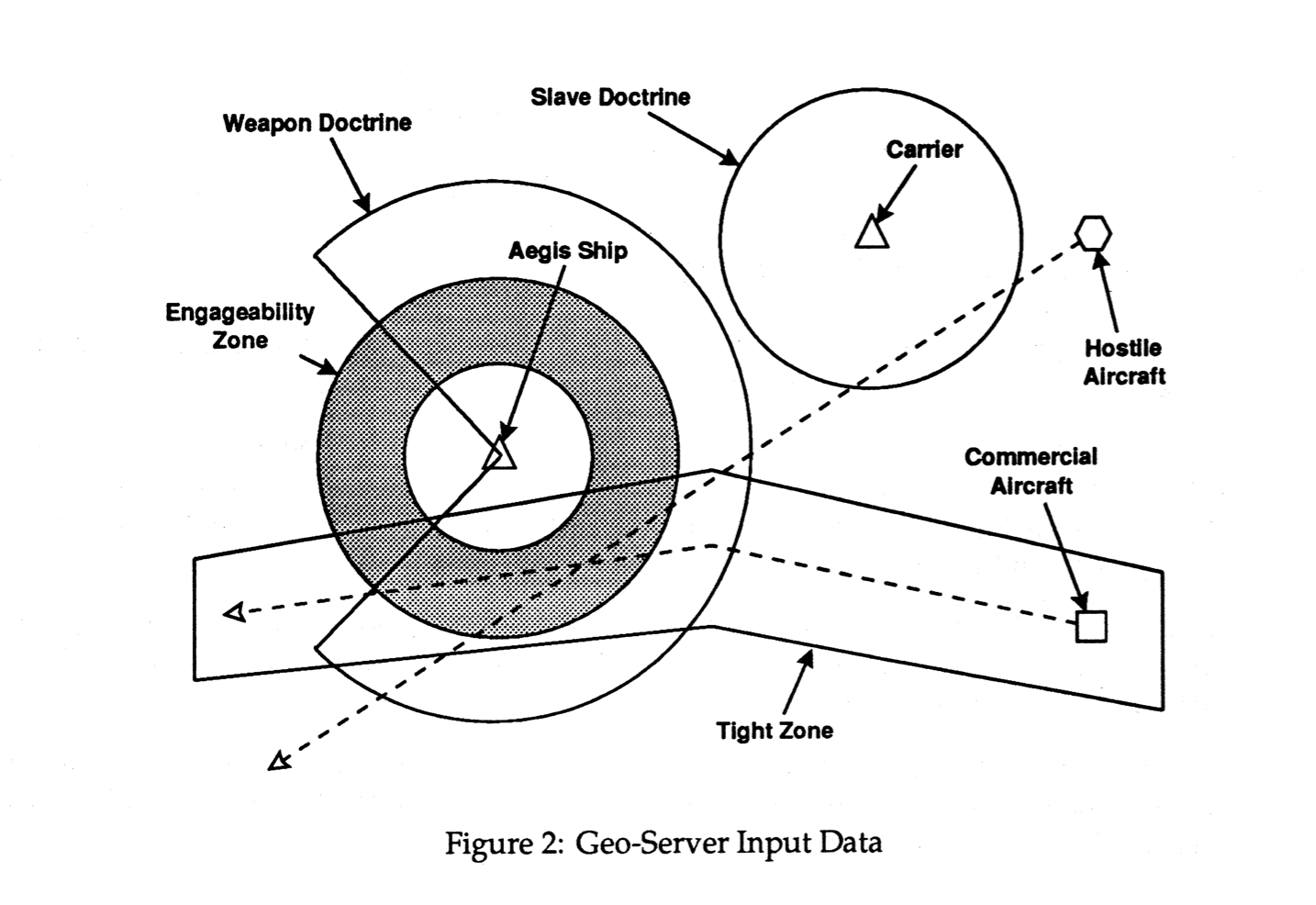## Shape Language

• Describe simple and compound shapes.
• Check if a point is inside a a shape.

## Shape Language

``````
(inside? some-shape some-point) ;; => true | false
``````

## Shape Language

1. Primitives
2. Means of combination
3. Means of abstraction

## Primitives

``````
(point x y)   ;; x & y are coordinates

circle        ;; unit circle centered at the origin
``````

## Combination

``````
;; All functions return a new shape

(translate shape dx dy)

(scale shape factor)

(union a b ...)

(intersection a b ...)

``````

## Abstraction

### Clojure!

This is an embedded DSL.

## Implementation

• Outside in
• Closure
• Syntactic sugar

### Outside In

``````
(defn point [x y] (->Point x y))

(def origin (point 0 0))

(def circle (->Circle origin 1))
``````

### Outside In

``````
(defprotocol Shape
(inside? [this other])
(scale [this s])
(translate [this dx dy]))
``````
``````
(defrecord Point [x y]
Shape
(inside? [this other]
(and (instance? Point other)
(= this other)))
(scale [_ s]
(->Point (* x s)
(* y s)))
(translate [_ dx dy]
(->Point (+ x dx)
(+ y dy))))
``````

### Closure

``````
;; Define a circle centered at (4, 3) with a radius of 5

(def some-circle
(-> circle
(scale 5)
(translate 4 3)

;; origin must be inside this shape

(inside? some-circle origin) ;; => true
``````

### Syntactic Sugar

``````
(->Point x y)  ;; concrete, Point record
``````

vs.

``````
(point x y)    ;; abstract shape
``````

### Syntactic Sugar

``````
(def list-and-an-item
(gen/bind (gen/not-empty (gen/vector gen/byte))
(fn [xs] (gen/tuple (gen/elements xs)
(gen/return xs)))))
``````

vs.

``````
(def list-and-an-item-2
(gen/let [xs (gen/not-empty (gen/vector gen/byte))
elem (gen/elements xs)]
[elem xs]))
``````

clojure.test.check.generators/let

### Optimizations

• Shape simplification
• Transform elimination
• Use transform matrix

## Thanks!

muhuk.github.io/clojure-dsl-presentation

### Appendix: Images